# Irregular Types of Linear Programming Problems

[Page 53 ( continued )]

The basic forms of typical maximization and minimization problems have been shown in this chapter. However, there are several special types of atypical linear programming problems. Although these special cases do not occur frequently, they will be described so that you can recognize them when they arise. These special types include problems with more than one optimal solution, infeasible problems, and problems with unbounded solutions.

For some linear programming models, the general rules do not always apply .

#### Multiple Optimal Solutions

Consider the Beaver Creek Pottery Company example, with the objective function changed f rom Z = 40 x 1 + 50 x 2 to Z = 40 x 1 + 30 x 2 :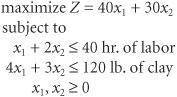where

x 1 = bowls produced

x 2 = mugs produced

The graph of this model is shown in Figure 2.20. The slight change in the objective function makes it now parallel to the constraint line, 4 x 1 + 3 x 2 = 120. Both lines now have the same slope of 4/3. Therefore, as the objective function edge moves outward from the origin, it touches the whole line segment BC rather than a single extreme corner point before it leaves the feasible solution area. This means that every point along this line segment is optimal (i.e., each point results in the same profit of Z = \$1,200). The endpoints of this line segment, B and C , are typically referred to as the alternate optimal solutions. It is understood that these points represent the endpoints of a range of optimal solutions.

Alternate optimal solutions are at the endpoints of the constraint line segment that the objective function parallels .

Multiple optimal solutions provide greater flexibility to the decision maker .

The pottery company, therefore, has several options in deciding on the number of bowls and mugs to produce. Multiple optimal solutions can benefit the decision maker because the number of decision options is enlarged. The multiple optimal solutions (along the line segment BC in Figure 2.20) allow the decision maker greater flexibility. For example, in the case of Beaver Creek Pottery Company, it may be easier to sell bowls than mugs; thus, the solution at point C , where only bowls are produced, would be more desirable than the solution at point B , where a mix of bowls and mugs is produced.

[Page 54]
##### Figure 2.20. Graph of the Beaver Creek Pottery Company example with multiple optimal solutions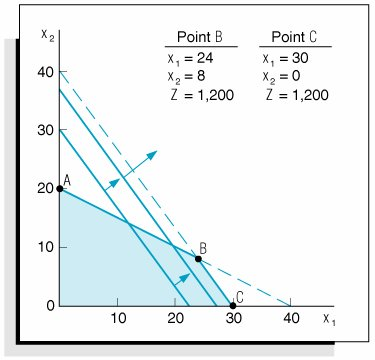#### An Infeasible Problem

In some cases a linear programming problem has no feasible solution area; thus, there is no solution to the problem. An example of an infeasible problem is formulated next and depicted graphically in Figure 2.21: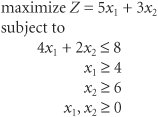##### Figure 2.21. Graph of an infeasible problem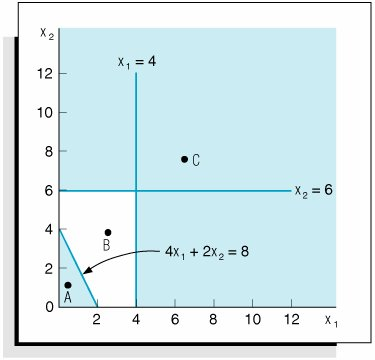An infeasible problem has no feasible solution area; every possible solution point violates one or more constraints .

[Page 55]

Point A in Figure 2.21 satisfies only the constraint 4 x 1 + 2 x 2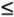8, whereas point C satisfies only the constraints x 1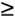4 and x 26. Point B satisfies none of the constraints. The three constraints do not overlap to form a feasible solution area. Because no point satisfies all three constraints simultaneously , there is no solution to the problem. Infeasible problems do not typically occur, but when they do, they are usually a result of errors in defining the problem or in formulating the linear programming model.

#### An Unbounded Problem

In some problems the feasible solution area formed by the model constraints is not closed. In these cases it is possible for the objective function to increase indefinitely without ever reaching a maximum value because it never reaches the boundary of the feasible solution area.

In an unbounded problem the objective function can increase indefinitely without reaching a maximum value.

An example of this type of problem is formulated next and shown graphically in Figure 2.22: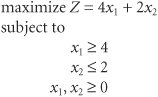##### Figure 2.22. An unbounded problem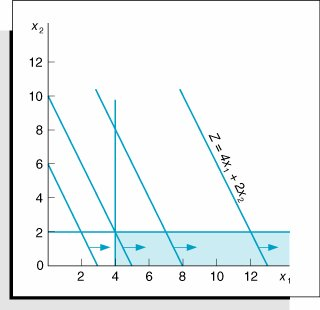In Figure 2.22 the objective function is shown to increase without bound; thus, a solution is never reached.

The solution space is not completely closed in .

Unlimited profits are not possible in the real world; an unbounded solution, like an infeasible solution, typically reflects an error in defining the problem or in formulating the model.Introduction to Management Science (10th Edition)
ISBN: 0136064361
EAN: 2147483647
Year: 2006
Pages: 358

Similar book on Amazon

flylib.com © 2008-2017.
If you may any questions please contact us: flylib@qtcs.net